×

×

# In each part, determine whether the equation is linear in , , and . (a) (b)ISBN: 9780470432051 396

## Solution for problem 1 Chapter 1.1

Elementary Linear Algebra: Applications Version | 10th Edition

• Textbook Solutions
• 2901 Step-by-step solutions solved by professors and subject experts
• Get 24/7 help from StudySoup virtual teaching assistantsElementary Linear Algebra: Applications Version | 10th Edition

4 5 1 402 Reviews
29
3
Problem 1

In each part, determine whether the equation is linear in , , and . (a) (b)

Step-by-Step Solution:

Problem-1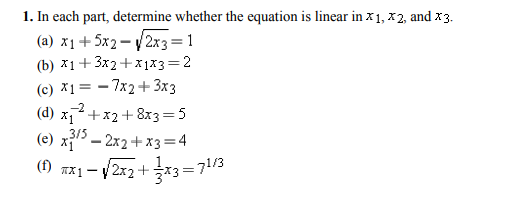Step-by-step solution

A linear equation in the n variables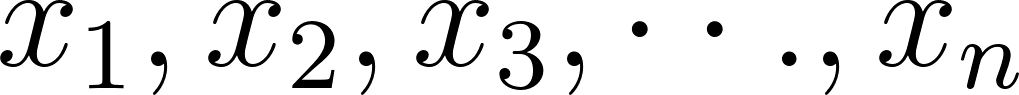to be one that can expressed in the formWhere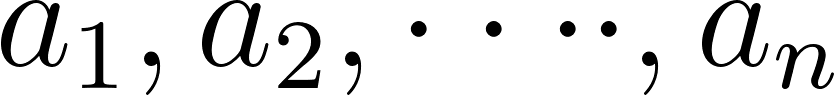and b are real constants .

Step 1 of  6

(a) Consider the equation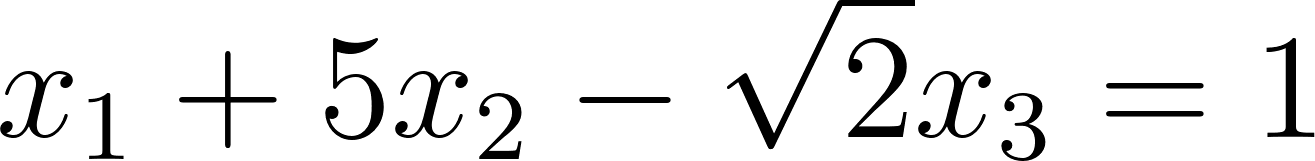It is needed to determine whether the equation is linear or not inand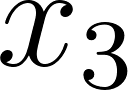.

Observe the equationdoes not involve any products or roots of the variables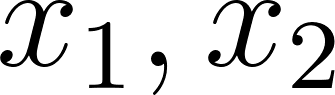and.

It is in the form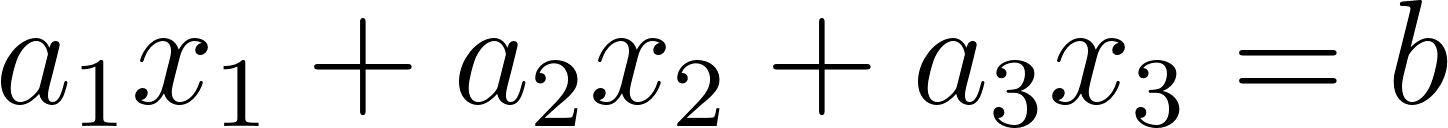with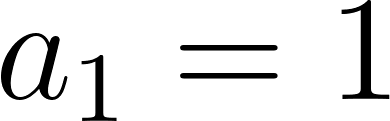,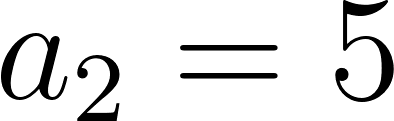,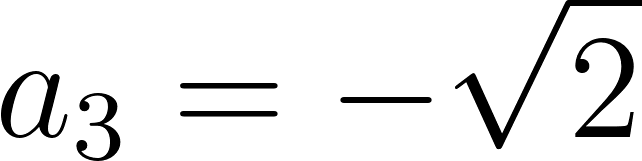and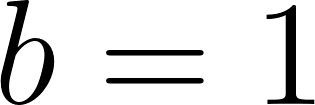which are all real constants .

All variables occur only to the first power and do not appear as arguments for trigonometric,logarithmic,or exponential functions.

Therefore,the equationis a linear equation.

Step 2 of 6

Step 3 of 6

##### ISBN: 9780470432051

The answer to “In each part, determine whether the equation is linear in , , and . (a) (b)” is broken down into a number of easy to follow steps, and 16 words. This textbook survival guide was created for the textbook: Elementary Linear Algebra: Applications Version, edition: 10. Since the solution to 1 from 1.1 chapter was answered, more than 255 students have viewed the full step-by-step answer. Elementary Linear Algebra: Applications Version was written by and is associated to the ISBN: 9780470432051. The full step-by-step solution to problem: 1 from chapter: 1.1 was answered by , our top Math solution expert on 03/13/18, 08:29PM. This full solution covers the following key subjects: . This expansive textbook survival guide covers 83 chapters, and 2248 solutions.

Unlock Textbook Solution